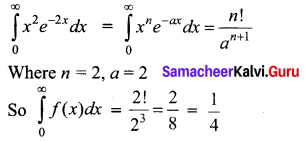# Samacheer Kalvi 12th Business Maths Solutions Chapter 2 Integral Calculus I Ex 2.10

Students can download 12th Business Maths Chapter 2 Integral Calculus I Ex 2.10 Questions and Answers, Samacheer Kalvi 12th Business Maths Book Solutions Guide Pdf helps you to revise the complete Tamilnadu State Board New Syllabus and score more marks in your examinations.

## Tamilnadu Samacheer Kalvi 12th Business Maths Solutions Chapter 2 Integral Calculus I Ex 2.10

Question 1.
Evaluate the following.

(i) Γ(4)
Solution:
Γ(4) = Γ(3 + 1) = 3! = 6

(ii) Γ($$\frac{9}{2}$$)
Solution: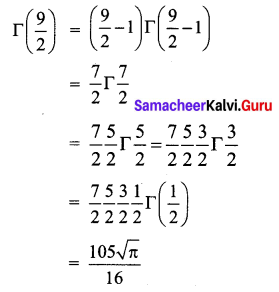(iii) $$\int_{0}^{\infty} e^{-m x} x^{6} d x$$
Solution: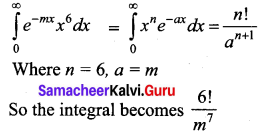(iv) $$\int_{0}^{\infty} e^{-4 x} x^{4} d x$$
Solution: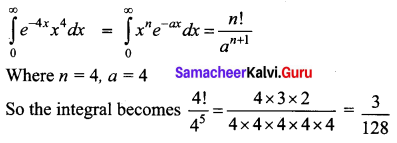(v) $$\int_{0}^{\infty} e^{-\frac{x}{2}} x^{5} d x$$
Solution: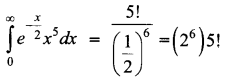Question 2.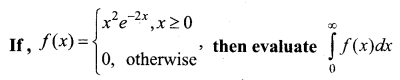Solution: